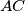« Vrati se

#### IMO Shortlist 2008 problem G6

There is given a convex quadrilateral$ABCD$. Prove that there exists a point$P$ inside the quadrilateral such thatif and only if the diagonals$AC$ and$BD$ are perpendicular.

Proposed by Dukan Dukic, Serbia